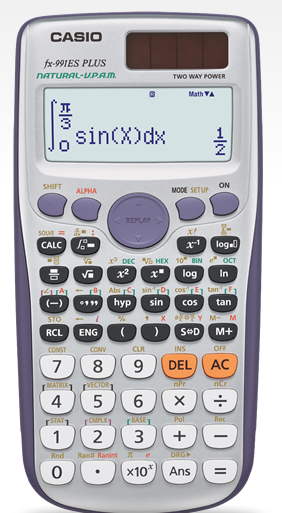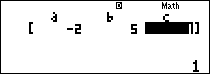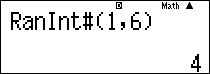# Casio FX-991ES Plus Scientific Calculator with 417 Functions #C58B*Image may differ with actual product's layout, color, size & dimension. No claim will be accepted for image mismatch.

# Casio FX-991ES Plus Scientific Calculator with 417 Functions #C58B

Product Id: 130.01.685.09

Regular Price  1,630
Special Price  1,530

Quick Overview

Model - Casio FX-991ES Plus
Brand - Casio
Type - Scientific Calculator
Display - Natural Textbook Dot Matrix Display
Digit - 10+2 Digits Display
Key - Plastic keys

### This item is currently unavailable

Model
Casio FX-991ES Plus
Type
Scientific Calculator
Display
Natural Textbook Dot Matrix Display
Digit
10+2 Digits Display
Key
Plastic keys
Number of Functions
417 Functions
Power Sources
Solar & Battery
Dimensions
161.5 x 77 x 11.1mm
Weight (Kg)
95gm
Specialty
New equation mode, Random integers, Fraction calculations, Combination and permutation, Statistics (List-based STAT data editor, standard deviation, regression analysis), 9 variables, Table function, Comes with new slide-on hard case, Equation calculations, Integration/differential calculations, Matrix calculations, Vector calculations, Complex number calculations, CALC function, SOLVE function, Base-n calculation, can create a numerical table based on that expression by registering a function expression, In addition, graph functions can be represented by graphs, Scientific Constants, can use constant symbols used in physics, science classes, Metric conversion function, can convert a number in one unit to a number in a different unit, List based STAT-data editor, Viewing and editing of input data in list format, showing data groups (x-data, y-data, frequency) and surrounding data, Differentiation, Non Graphing, Matrix, Equation, Integration, Vector, Complex number calculation
Others
Part no: C58B, Natural textbook display: Display expression same as textbook, 10 + 2 digits: 10-digit mantissa + 2-digit exponential display, Dot matrix display: High-resolution screen provides beautiful looking graphs every time, Multi-replay: Quick and easy recall of previously executed formulas for editing and re-execution, Solar & Battery: Solar powered when light is sufficient, battery powered when light is insufficient, Plastic keys: Designed and engineered for easy operation, Basic Statistics: Standard statistics functions such as Mean, SUM, Standard Deviation, and Regression, Base-n calculation: Binary/Octal/Hexadecimal, Basic Mathematical Functions: Trigonometric, Exponential logarithmic, etc.
Warranty
3 year
Thailand
Country Of Origin
Japan

# Casio FX-991ES Plus# Software features

### New equation mode (√and fraction display)Random integers## Other features

• Two way power
• 417 Functions
• Full Dot Display
• Plastic Keys
• Integration / Differential
• Equation calculation
• Matrix calculations / Vector calculations
• Complex number calculation
• CALC function / SOLVE function
• Base-n calculation
• Fraction calculation
• Combination and Permutation
• Logarithm log
• List-based STAT data editor
• Standard deviation
• Paired-variable statistics regression analysis
• Logical operations
• Table function
• 40 scientific constants
• 40 metric conversions (20 conversion pairs)
• 9 variable memories
• Comes with new slide on hard case

Model - Casio FX-991ES Plus, Brand - Casio, Type - Scientific Calculator, Display - Natural Textbook Dot Matrix Display, Digit - 10+2 Digits Display, Number of Functions - 417 Functions, Key - Plastic keys, Power source - Solar & Battery, Dimension - 161.5 x 77 x 11.1mm, Weight - 95gm, Others - Part no: C58B, Natural textbook display: Display expression same as textbook, 10 + 2 digits: 10-digit mantissa + 2-digit exponential display, Dot matrix display: High-resolution screen provides beautiful looking graphs every time, Multi-replay: Quick and easy recall of previously executed formulas for editing and re-execution, Solar & Battery: Solar powered when light is sufficient, battery powered when light is insufficient, Plastic keys: Designed and engineered for easy operation, Basic Statistics: Standard statistics functions such as Mean, SUM, Standard Deviation, and Regression, Base-n calculation: Binary/Octal/Hexadecimal, Basic Mathematical Functions: Trigonometric, Exponential logarithmic, etc., Specialty - New equation mode, Random integers, Fraction calculations, Combination and permutation, Statistics (List-based STAT data editor, standard deviation, regression analysis), 9 variables, Table function, Comes with new slide-on hard case, Equation calculations, Integration/differential calculations, Matrix calculations, Vector calculations, Complex number calculations, CALC function, SOLVE function, Base-n calculation, can create a numerical table based on that expression by registering a function expression, In addition, graph functions can be represented by graphs, Scientific Constants, can use constant symbols used in physics, science classes, Metric conversion function, can convert a number in one unit to a number in a different unit, List based STAT-data editor, Viewing and editing of input data in list format, showing data groups (x-data, y-data, frequency) and surrounding data, Differentiation, Non Graphing, Matrix, Equation, Integration, Vector, Complex number calculation, Warranty - 3 year, Country of Origin - Japan, Made in/ Assemble - Thailand